# Pi goes on forever

In honor of Pi Day — 3.14.2011 — we offer a brief history of Pi and of its computation.

By David H. Bailey and Jonathan M. Borwein

I. A brief history of Pi

The mathematical constant we now know as Pi = 3.14159… has fascinated mathematicians for millennia. Archimedes of Syracuse (~250 BCE) rigorously showed that the area of a circle is Pi times the square of the radius. He then presented an approximation scheme, based on inscribed and circumscribed polygons, which enabled one to compute Pi to any desired accuracy. He himself found, with laborious and ingenious computation, that 3 10/71 < Pi < 3 1/7, so that 3.1408 < Pi < 3.1428.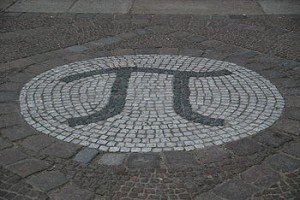Figure 1. Pi in tiles outside the Matheon research centre in Berlin

in ancient times was indeed very difficult, until the discovery, in fifth century India, of our modern positional decimal arithmetic system with zero. It took nearly 1000 years for this system to be widely adopted in Europe, but when it did, mathematicians, armed with advances such as calculus, discovered countless new formulas and other facts involving Pi, and computed numerical values of Pi with great aplomb. Isaac Newton computed Pi to at least 15 digits, in the plague year 1666, although he sheepishly acknowledged “I am ashamed to tell you how many figures I carried these computations, having no other business at the time.”

Leonhard Euler (1707-1783), arguably the most prolific mathematician in history, discovered several new formulas for Pi, one of which later led German mathematician Bernhard Riemann (1826-1866) to present what is now known as the Riemann zeta function hypothesis, an unproven conjecture with rich connections to many fields of mathematics. The Clay Mathematics Institute in the U.S. has offered an award of \$1,000,000 for a proof.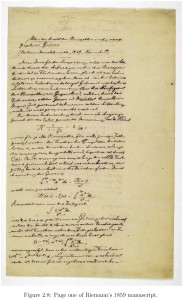Figure 2: Riemann writes on the Riemann zeta function

Carl Friedrich Gauss (1777-1855) discovered many interesting connections between Pi and applied mathematics and physics. Among these many discoveries was the essential idea of a clever computational technique, now known as the “fast Fourier transform,” which intimately involves Pi. This scheme is very heavily utilized in audio, video, microwave and data transmission applications. Cell phones typically include at least one special-purpose chip to perform this operation. Gauss also discovered some interesting facts regarding the “arithmetic-geometric mean”, which (see below) later led to a new algorithm for calculating Pi.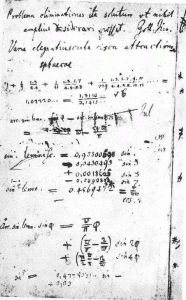Figure 3: Gauss and the arithmetic-geometric mean

Some progress, but not a great deal, was made in computing Pi during this time. At the end of the Reformation in 1648, Pi had been calculated only to 35 digits; even by the end of World War II in 1945 it was known to only 527 digits. In 1948 ENIAC, the first digital computer, computed 2037 digits, and the race was on.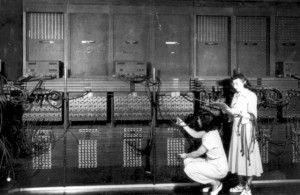Figure 4. Programming on ENIAC in 1946

II. Pi in the computer age

In 1965, computer scientists rediscovered Gauss’ fast Fourier transform technique, and noted that among things it could be used to greatly accelerate many-digit multiplication operations. Also in 1965, Gordon E. Moore, one of the founders of Intel, noted that because of advances in silicon semiconductor technology, the number of transistors that could be placed on a single chip had roughly doubled each year since 1960, and, from what he could see, this trend was likely to continue for several more years. Much to the astonishment of everyone in the field, “Moore’s Law,” as this observation is now known, has continued unabated for more than 45 years, and no end is yet in sight. As a result, a present-day laptop computer has more computing power and memory storage than the world’s most powerful supercomputer of just 20 years ago, and present-day high-end supercomputers (which typically are highly parallel arrays of commodity components) are many thousands of times more powerful and capacious than today’s laptops.

A few years later, mathematicians Richard Brent (at the Australian National University) and Eugene Salamin independently discovered a way to compute Pi, based on some results by Gauss on the arithmetic-geometric mean and lovely classical ideas about elliptic integrals. This yielded a much faster scheme than the traditional calculus-based formulas for Pi — with each step, this new algorithm doubles the number of correct digits (provided that one is using computer arithmetic that is sufficiently accurate).

Armed with these new formulas, numerical techniques and rapidly growing computing power, scientists computed Pi to over one million digits in 1973, to over one billion digits in 1989, and then to over one trillion digits in 2009. The most recent computation (August 2010) was done by Alexander Yee and Shigeru Kondo, who computed five trillion digits of Pi. Remarkably, this was not done on a highly parallel supercomputer, but instead was performed on a conventional two-core Intel Xeon computer equipped with 96 Gbyte of main memory. They employed a remarkable sum formula for Pi due to David and Gregory Chudnovsky, and checked their results using variants of a new formula for Pi discovered in 1996 by Peter Borwein (Jonathan Borwein’s brother), Simon Plouffe and David Bailey. This new formula for Pi has the odd property that it permits one to calculate binary (base-2 or base-16) digits of Pi beginning at an arbitrary starting position, without needing to calculate any of the digits that came before. This scheme was itself employed in 2010 to calculate binary digits beginning at position two quadrillion (by the way, the two quadrillionth binary digit of Pi is a 0).

For Pi day 2011, the authors along with Andrew Mattingly and Glenn Wightwick of IBM Australia computed digits of various constants in various bases on an IBM blue Gene/P machine. The results include base-64 digits of ?? beginning at position 10 trillion, which in base-eight are: 60114505303236475724500005743262754530363052416350634.
This suite of computations in total took roughly 1200 years of serial computing time (roughly 110 “rack days”).Figure 5. Kondo and Ye with their 2010 home computer

It is amusing to note that as recently as 1961, Daniel Shanks, who himself calculated Pi to over 100,000 digits, declared that computing one billion digits would be “forever impossible.” This was achieved in 1989 by Yasumasa Kanada of Japan. And in 1989, noted British physicist Roger Penrose, in the first edition of his best-selling book The Emperor’s New Mind, declared that we likely will never know if a string of ten consecutive sevens occurs in the decimal expansion of Pi. This string was found just eight years later (1997), also by Kanada, beginning at position 22,869,046,249.Figure 6. A rack of an IBM Blue Gene

III. Why calculate digits of Pi?

Certainly there is no need for computing Pi to millions or billions of digits in practical scientific or engineering work. A value of Pi to 40 digits would be more than enough to compute the circumference of the Milky Way galaxy to an error less than the size of a proton. There are certain scientific calculations that require intermediate calculations to be performed to significantly higher precision than required for the final results, but it is doubtful than anyone will ever need more than a few hundred digits of Pi for such purposes. Values of Pi to several thousand digits are sometimes employed in “experimental” mathematics, but very few such computations require Pi to beyond 25,000 digits, and we are not aware of any requiring Pi to more than 10 million digits.

One practical application for computing digits of Pi is that these calculations are excellent tests of the integrity of computer hardware and software. If two separate computations of digits of Pi, say using different algorithms, are in agreement except perhaps for a few trailing digits at the end, then almost certainly both computers performed trillions of operations flawlessly. For example, in 1986, a Pi-calculating program that David Bailey wrote at NASA, using an algorithm due to Jonathan and Peter Borwein, detected some hardware problems in one of the original Cray-2 supercomputers that had escaped the manufacturer’s tests.

Along this same line, some improved techniques for computing the fast Fourier transform on modern computer systems had their roots in efforts to accelerate computations of Pi. These improved techniques are now very widely employed in scientific and engineering applications.

From a mathematical point of view, one historical motivation for computing Pi was to see if the digits of Pi repeat, thus disclosing that Pi is a simple ratio of two integers. But this question was laid to rest in the 1760s, when Lambert and later Legendre used the theory of continued fractions to prove that Pi cannot be written as the ratio of two integers. The more general question of whether Pi is the solution to an algebraic equation (to be specific, the root of a polynomial with integer coefficients) was laid to rest in 1882, when Lindemann proved that Pi is not of this class.

There are many interesting mathematical questions that remain. In fact, we can prove shockingly little about either the digits of Pi or about its continued fraction. The most notable open question is probably this: Are the digits of Pi truly “random” in a statistical sense? This is more formally stated as the question of whether Pi is a “normal” number. A normal number is one whose digits in some number base (say base 10) have the property that the frequency of appearance of any finite-length string (say “4567”) tends to the expected frequency (1 in 10,000 in this case) as more and more digits are tabulated. It is widely believed that Pi has this property, not just for decimal digits but for all number bases. However, no one has been able to prove this assertion, and so mathematicians have analyzed large numbers of digits looking for any indication that this property might not hold, or for clues on how to rigorously analyze this question. A solid proof of this conjecture would yield, for instance, a provable source of pseudo-random numbers for computer-based statistical investigations. Until such a proof is in hand, it is always possible that a much longer calculation will indicate that Pi is not normal. Figure 4 shows how digit patterns in the fractions (22/7 and 223/71) jump out, while no such pattern is discernable in 100 digits of Pi (center).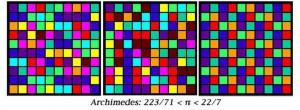Figure 7. A colour calculator

But there is a more fundamental motivation for computing Pi, which should be familiar to anyone who has scaled a lofty mountain or competed in a major sporting event: “because it is there”– it is easily the most famous mathematical constants, and its properties have fascinated mathematicians and other scientists for millennia. Thus, as long as there are humans (and computers) we will doubtless witness ever-more impressive computations of Pi.

Happy birthday, Pi!

This material and much more can be followed up at The Life of Pi lecture for Pi Day 2011: JMB PiDay talk, a book chapter on Pi: JMB Pi chapter, an article on Pi in “The Conversation”: Conversation, and at David Bailey’s Pi Resources: DHB Pi site. Readers may also be amused by the following music set to the digits of Pi: Musical Pi.